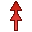# A6 Card

This card specifies excitation by an elementary magnetic Hertzian dipole.

On the Source/Load tab, in the Ideal sources group, click theMagnetic dipole (A6) icon.

## Parameters:

New source
A new excitation is defined which replaces all previously defined excitations.
A new excitation is defined which is added to the previously defined excitations.
Electric ring current
Use the model of an electric ring current for the magnetic dipole (the moment $m=µIA$ where I is the loop current and A the enclosed surface area).
Magnetic line current
Use the model of an magnetic line current (the moment $m=\frac{{I}_{m}l}{j\omega }$ where Im is the magnetic line current and $l$ is the length of the dipole).
Magnitude of I A (A m^2)
The magnitude of the dipole. For the electric ring current it is $I\cdot A$ in Am2. For the magnetic line current it is ${I}_{m}\cdot l$ in Vm.
Phase (degrees)
Phase of the complex amplitude in degrees.
X, Y, Z position

Coordinates of the position of the dipole in m. These values are optionally scaled by the SF card.

$\vartheta$ angle
The angle between the dipole and the Z axis in degrees.
$\phi$ angle

The angle between the projection of the dipole onto the plane Z=0 and the X axis in degrees.

The power radiated by the dipole in a free space environment is given by
(1) $p=\frac{{\beta }_{0}^{4}{Z}_{F0}{|IA|}^{2}}{12\pi }$

Feko, however, considers the properties of the medium in which the dipole is located, as well as the coupling of the dipole with surrounding structures or other sources (for example other magnetic dipoles in an array antenna), when calculating the power radiated by the Hertzian dipole.

Even though the two formulations, electric ring current and magnetic dipole, result in the same near and far fields, if the dipole moment m is the same, the radiated potentials are different.

The electric ring current model gives rise to a magnetic vector potential A while the magnetic dipole model results in an electric vector potential F as well as a magnetic scalar potential $\psi$ .